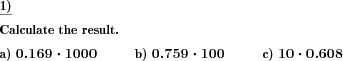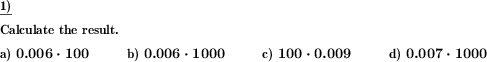Custom math worksheets at your fingertips# Details for problem "Decimal fraction multiply by power of 10"

Quickname: 8565

Elementary School, Primary School, Junior High School, Middle School, High School.

## Summary

A decimal fraction has to be multiplied by a power of ten.

## Examples## Description

A decimal fraction is one of the basic topics to learn in Maths and this worksheet provides practice problems related to decimal fractions multiplied with a power of 10. A decimal fraction is presented, it is one factor in a multiplication problem. The other factor is a power of ten. Practicing these worksheets is necessary for learners to grasp multiplication with decimal fractions skills. Assign these worksheets to struggle students for independent practice and let them grasp the skills. These can also be used in variation for assessment purposes. Along with this main objective, grade 6 to 8 learners will also achieve the following set of targets.

1. Revise the concepts of multiplication.
2. Reinforce the concept of multiplication with a power of 10.
3. Use mental maths times table skills.
4. Master the multiplication of a decimal fraction with a power of 10.
5. Apply this skill to real-world problems.

Personalize these problems and serve to differentiated ability learners.

• The number of places before the decimal point can be selected.
• The number of decimals can be selected for the decimal fraction factor.
• In addition, the first n decimal places can be made with zero digits. In this way, several different shapes can be generated.
• For the other factor, the maximum power of ten applied can be defined.

Some examples:

Number of places: 0 Number of decimals: 3 Leading decimals=0: 0

```    0.222 * 100      0.456 * 1000      0.999 * 10
```

Number of places: 2 Number of decimals: 2 Leading decimals=0: 0

```    12.21 * 100      89.12 * 1000      23.44 * 10
```

Number of places: 0 Number of decimals: 3 Leading decimals=0: 2

```    0.002 * 100      0.001 * 1000      0.008 * 10
```

The number of problems can be chosen. The answer key consists of all problems with solutions.

Download free printable worksheets for this math problem here. The worksheet contains the problems only, the solution sheet includes the answers. Just click on the respective link.

•Worksheet 1Solution sheet with answers
•Worksheet 2Solution sheet with answers
•Worksheet 3Solution sheet with answers

If you can not see the solution sheets for download, they may be filtered out by an ad blocker that you may have installed. If this is the case, please allow ads for this page and reload the page. The solution sheets will then reappear.

• Do these sample worksheets do not really fit?
• Do you need more math worksheets, with a different level of difficulty?
• Would you like to combine different problems on a worksheet and adjust them to your needs?
• As a teacher, you can put together your own worksheets using the automatically generated math problems provided.
With a free initial credit, you can start creating your own math worksheets in a few minutes.

You can try it for free! Register here, to create custom worksheets now!

## Customization options for this problem

Parameter
Possible values
Number of problems
1, 2, 3, 4, 5, 6, 7, 8, 9, 10
Places
0, 1, 2, 3
Decimal places
1, 2, 3, 4
Decimals 0 up to pos
0, 1, 2, 3
Power of 10 up to
10, 100, 1000, 10000, 100000, 1000000
Swap factors
Yes, No